# Tutorials#

Learn how pyGIMLi can be used for modelling and inversion. The listed tutorials with increasing complexity start with basic functionality such as mesh generation and visualization and dive into the generalized modelling and inversion concepts including managers and frameworks. Check out the Examples for more specialized demonstrations and interesting case studies.

## Basics#

These introductory tutorials cover basic tasks such as meshes, data container, matrices, visualization, and more.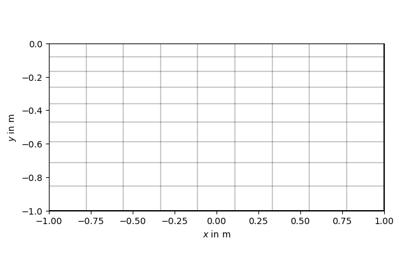GIMLi Basics

GIMLi BasicsThe DataContainer class

The DataContainer class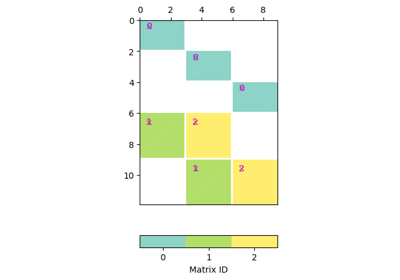Matrices

Matrices

## Meshes#

These introductory tutorials cover meshes from simple generation, their elements, visualization, interpolation and more.The anatomy of a pyGIMLi mesh

The anatomy of a pyGIMLi mesh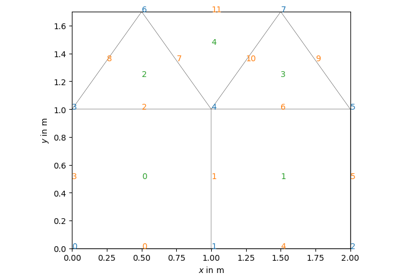The mesh class

The mesh class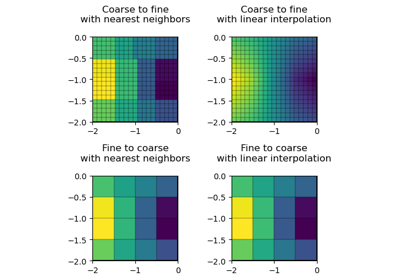Mesh interpolation

Mesh interpolation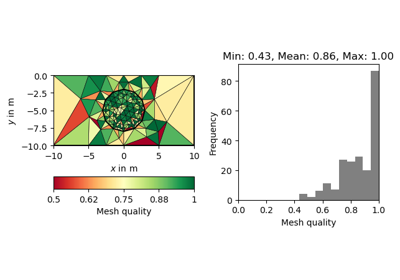Quality of unstructured meshes

Quality of unstructured meshesRegion markers

Region markers

## Modelling#

Here we collect examples and user stories that illustrate the modelling part of pygimli.Basics of Finite Element Analysis

Basics of Finite Element Analysis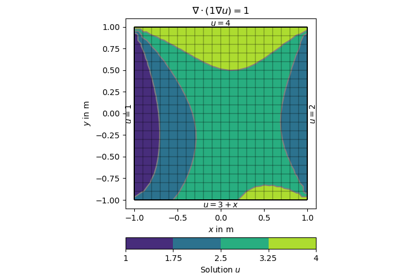Modelling with Boundary Conditions

Modelling with Boundary ConditionsHeat equation in 2D

Heat equation in 2D

## Inversion#

Simple inversions with increasing complexity.Simple fit

Simple fitPolyfit

PolyfitVES inversion for a blocky model

VES inversion for a blocky model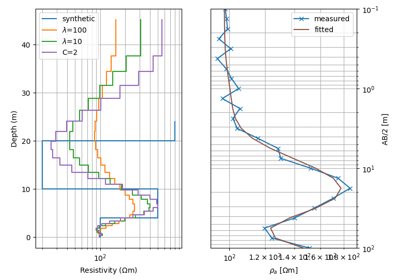VES inversion for a smooth model

VES inversion for a smooth modelRegularization - concepts explained

Regularization - concepts explained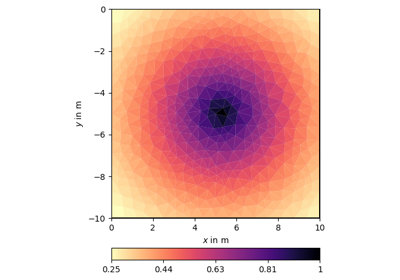Geostatistical regularization

Geostatistical regularization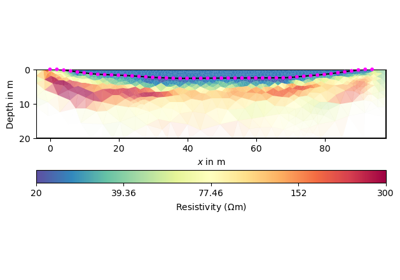Region-wise regularization

Region-wise regularization

Gallery generated by Sphinx-Gallery